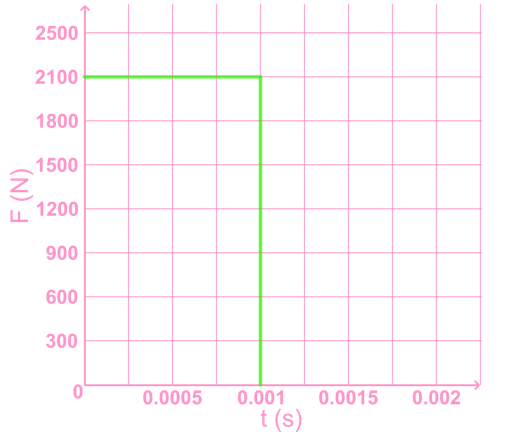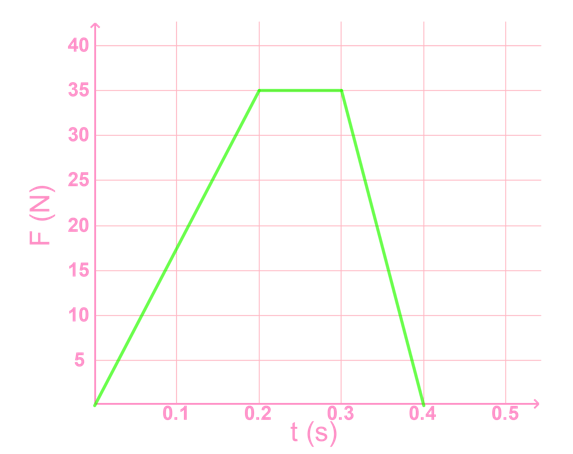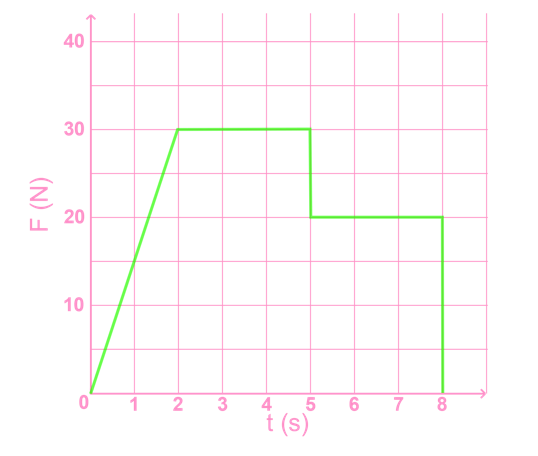# Momentum and impulse

### Momentum and impulse

#### Lessons

In this lesson, we will learn:

• What is impulse?
• Derivation of impulse from Newton's second law
• Impulse equations, units
• Problem solving with impulse and momentum
• Problem solving with F vs t graph

Notes:

• Impulse is change in an object's momentum.
• To change an object's momentum, an impulse must be given to that object. An example would be kicking a soccer ball (an impulse) to set it into motion (give it momentum).
• An impulse is defined as a force that acts on an object for a period of time.
• In a graph of force vs. time, the area under the graph is impulse.

Momentum

$\vec{p} = m \vec{v}$

$\vec{p}:$ momentum, in kilogram meters per second (kg∙m/s)

$m:$ mass, in kilograms (kg)

$\vec{v}:$ velocity, in meters per second (m/s)

Impulse

$\vec{J} = \vec{F} \Delta t = \Delta \vec{p} = m( \vec{v}_f - \vec{v}_i$)

$\vec{J}:$ impulse, in newton seconds (N∙s)

$\vec{F}:$ net force acting on an object, in newtons (N)

$\Delta t:$ the length of time for which the force acts, in seconds (s)

$\Delta \vec{p}:$change in momentum of an object, in kilogram meters per second (kg∙m/s)

• Introduction
Introduction to impulse and momentum:
a)
What is impulse?

b)
Derivation of impulse from Newton's second law

c)
Impulse equations, units

• 1.
Impulse = $\bold{\vec{F}}$$\bold{\Delta t = \Delta \vec{p}}$
a)
A tennis ball is initially at rest, then hit by a racket with 30.0 N [E]. The racket is in contact with the ball for 0.070 s. Find the impulse given to the ball by the racket, and the final momentum of the ball.

b)
A go kart has momentum 1000 kg·m/s forward when the engine applies a force of 55 N forwards for 6 s. Find the impulse given to the go kart by the engine and the final momentum of the go kart.

c)
A baseball is pitched with momentum 5.85 kg·m/s [S], and is hit by a bat which applies a force of 300 N [N]. The bat is in contact with the ball for 0.05 s. Find the impulse given to the ball and the final momentum of the ball.

• 2.
Impulse = $\bold{\vec{F}}$$\bold{\Delta t = m(\vec{v}_f - \vec{v}_i )}$
a)
A 1.25 kg skateboard rolls forward at 0.60 m/s. It is given a push forward with a force of 5.0 N for 1.2 s. What is the final velocity of the skateboard?

b)
A soccer ball is initially at rest. Kicking the ball with an impulse 1.30 N·s causes the ball to move at 3.00 m/s. What is the mass of the ball?

c)
A 0.035 kg rubber ball is thrown at a wall at 13 m/s and rebounds at 7 m/s. Find the impulse given to the ball by the wall.

d)
A 1200 kg car travels at 11 m/s [E]. At t = 5.0 s, the driver starts accelerating with a force of 300 N [E] applied to the car. If the final velocity of the car is 17 m/s, what is the time when the driver stops accelerating?

e)
A 50 kg ice skater glides without friction at 7.2 m/s [W] when he skates through a rough patch on the ice for 3.0 s. If his velocity after the patch is 4.5 m/s [W], what is the average force of friction experienced in the patch?

• 3.
Impulse of stopping a car

A 1300 kg car travelling at 13.5 m/s [E] can be stopped in 20 s with gentle braking, 4.3 s in a panic stop, and 0.78 s if it hits a wall.

1. What is the impulse given to the car to stop it in each case?
2. What is the average force experienced by the car in each case?

• 4.
Impulse = area under $\bold{F\;\mathrm{vs}\; t}$ graph, during the time interval $\bold{\Delta t}$
a)
A 0.060 kg golf ball is struck by a golf club which exerts the force shown in the graph over a period of 0.001 s in the forward direction. Find the impulse exerted on the ball, and the final velocity of the ball.b)
b) A 0.425 kg soccer ball is moving 0.80 m/s [E] when it is kicked and experiences the force shown in the graph below. The direction of the kick is [W]. Find the final velocity of the ball.c)
Two people begin pushing a cart. The force experienced by the cart is shown in the graph. At t = 5 s, person A lets go of the cart. At t = 12 s, person B lets go of the cart. Find the impulse person B gives the cart during the time he is the only person pushing it.• 5.
Momentum and Impulse in two dimensions
a)
a) A 1300 kg car travelling 12 m/s [W] is given a 1.6×104 N·s [N] impulse. What is the final velocity of the car?

b)
A 1.40 kg ball rolling 8.00 m/s [N] hits a barrier and bounces off, then moving 6.20 m/s [E]. What was the impulse given to the ball?

c)
A 0.25 kg billiard ball in motion is given an impulse of 0.78 N·s [E]. If the final velocity of the ball is 3.2 m/s [27° S of E], what was the initial velocity of the ball?